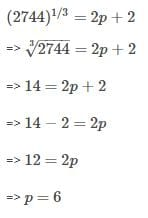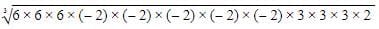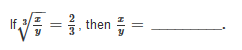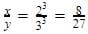# Olympiad Test: Cubes And Cube Roots

## 20 Questions MCQ Test UPSC Prelims Paper 2 CSAT - Quant, Verbal & Decision Making | Olympiad Test: Cubes And Cube Roots

Description
Attempt Olympiad Test: Cubes And Cube Roots | 20 questions in 20 minutes | Mock test for Class 8 preparation | Free important questions MCQ to study UPSC Prelims Paper 2 CSAT - Quant, Verbal & Decision Making for Class 8 Exam | Download free PDF with solutions
QUESTION: 1

### A natural number is said to be a perfect cube, if it is the cube of some _________.

Solution:

The cube of a natural number is always a natural number.

QUESTION: 2

### The cube of 23 is ___________

Solution:

23= 23×23×23 = 12167

QUESTION: 3

### Find the cube of 75.

Solution:
QUESTION: 4

If n = m × m × m = m3, where m is an integer, then n is a perfect cube and the number m is called the __________ of n.

Solution:
QUESTION: 5

If (2744)1/3 = 2p+2, then the value of P is

Solution:QUESTION: 6

The cube root of 13824 is __________.

Solution:
By prime factorisation:
13824 is (2x2x2)x(2x2x2)x(2x2x2)x(3x3x3)
Making pairs of three three numbers
which gives 2x2x2x3=24
Therefore cube root of 13824 is 24
QUESTION: 7

The square of a natural number subtracts from its cube comes 100. The number is __________.

Solution:
QUESTION: 8

Find the cube root of 0.001331.

Solution:
QUESTION: 9

Find the smallest number by which 54 must be multiplied so that the product is a perfect cube.

Solution:
QUESTION: 10

Find the ones digit of cube root of 2197.

Solution:

2197

Unit's place of 2197 = 7

The ones digit of cube root of 2197 will be 3 as 3= 27 which has 7 as unit's place

QUESTION: 11

Find the cube root of -5832.

Solution:
QUESTION: 12

The cube root of the 216 x (−32) x 54 is ________

Solution:

We have 216 x (-32) x 54.
Applying prime factorisation giveswhich gives  -(6 x 2 x 2 x 3)= -72.

QUESTION: 13

If x is ones digit and y is tens digit of a two digit number, then the cube of the number will be _________.

Solution:
QUESTION: 14

If 72×K is a perfect cube, find the value of K.

Solution:

72 = 2×2×2×3×3

If  K = 3, 72×K = 72 × 3 = 216 = 63

is a perfect cube.

QUESTION: 15

If (504 + p) is a perfect cube number, whose cube root is p, then p = ______.

Solution:

Dear Student, we know that 83= 512
So p = 512−504 = 8

QUESTION: 16

23 is a cube root of _________.

Solution:
QUESTION: 17

Find the side of the cubical box whose volume is 474.552 dm3.

Solution:
QUESTION: 18

If volume of cube is 4913cm³ then length of side of cube is

Solution:
QUESTION: 19

Divide 5673375 by the smallest number so that the product is perfect cube.

Solution:

The Prime Factors are:
3 x 3 x 3 x 5 x 5 x 5 x 41 x 41

In Exponential Form:
3^3 x 5^3 x 41^2
So we have to divide by 41^2

QUESTION: 20Solution:

Taking cube both the sides,Use Code STAYHOME200 and get INR 200 additional OFF Use Coupon Code